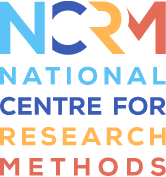# Structural Equation Modelling (SEM): What it is and what it isn't

## Presenter(s): Patrick SturgisIn this online learning resource, I will talk about the general structural equation modelling framework, the key terms and concepts in SEM and confirmatory factor analysis.

## Structural Equation Modeling: what is it and what can we use it for?

Strutural Equation Modelling is a framework which draws on a number of different disciplines it brings together measurement theory from psychology, factor analysis also from psychology and statistics, path analysis from epidemiology and biology, regression modeling from statistics and simultaneous equations from econometrics, and all these different techniques come together to form structural equation modeling as a general modeling environment. And it's also an environment which is somewhat dynamic. It is not set in stone at this point in time it is actually often integrating new ways of fitting models as the technique develop over time.

## Key ideas, terms and concepts in Structural Equation Modelling

In this video I'm going to talk about some of the key ideas terms and concepts in structural equation modeling. This is important because SEM is rather different to other areas of statistics some of the ideas that are important in understanding and applying SEM are quite unfamiliar and so it's important to have a grounding and familiarity with these ideas before we move on to other applications

## Confirmatory Factor Analysis

In this video we moved to understanding some of the applications of the actual model fitting that go on in structural equation modeling and this focuses particularly on confirmatory factor analysis.